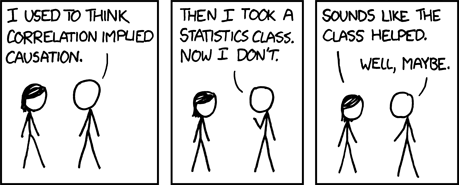## Recap and Proposal: 95/5, The Statistically Insignificant Privacy Guarantee

May 26th, 2010 by Mimi YinImage from: xkcd.

In our search for a privacy guarantee that is both measurable and meaningful to the general public, we’ve traveled a long way in and out of the nuances of PINQ and differential privacy: A relatively new, quantitative approach to protecting privacy. Here’s a short summary of where we’ve been followed by a proposal built around the notion of statistical significance for where we might want to go.

## The “Differential Privacy” Privacy Guarantee

Differential privacy guarantees that no matter what questions are asked and how answers to those questions are crossed with outside data, your individual record will remain “almost indiscernible” in a data set protected by differential privacy. (The corollary to that is that the impact of your individual record on the answers given out by differential privacy will be “negligeable.”)

For a “quantitative” approach to protecting privacy, the differential privacy guarantee is remarkably NOT quantitative.

So I began by proposing the idea that the probability of a single record being present in a data set should equal the probability of that single record not being present in that data set (50/50).

I introduced the idea of worst-case scenario where a nosy neighbor asks a pointed question that essentially reduces to a “Yes or no? Is my neighbor in this data set?” sort of question and I proposed that the nosy neighbor should get an equivocal (50/50) answer: “Maybe yes, but then again, (equally) maybe no.”

(In other words, “almost indiscernible” is hard to quantify. But completely indiscernible is easy to quantify.)

We took this 50/50 definition and tried to bring it to bear on the reality of how differential privacy applies noise to “real answers” to produce identity-obfuscating “noisy aswers.”

I quickly discovered that no matter what, differential privacy’s noisy answers always imply that one answer is more likely than another.My latest post was a last gasp explaining why there really is no way to deliver on the completely invisible, completely non-discernible 50/50 privacy guarantee (even if we abandoned Laplace).

(But I haven’t given up on quantifying the privacy guarantee.)

## Now we’re looking at statistical significance as a way to draw a quantitative boundary around a differential privacy guarantee.

Below is a proposal that we’re looking for feedback on. We’re also curious to know if anyone else tried to come up with a way to quantify the differential privacy guarantee?

## What is Statistical Significance? Is it appropriate for our privacy guarantee?

In statistics, a result is called statistically significant if it is unlikely to have occurred by chance. Applied to our privacy guarantee, you might ask the question this way: When you get an answer about a protected data set, are the implications of that “differentially private” answer (as in implications about what the “real answer” might be) significant or are they simply the product of chance?

Is this an appropriate way to define a quantifiable privacy guarantee, we’re not sure.

## Thought Experiment: Tossing a Weighted CoinYou have a coin. You know that one side is heavier than the other side. You have only 1 chance to spin the coin and draw a conclusion about which side is heavier.

At what weight distribution split does the result of that 1 coin spin start to be statistically significant?

Well, if you take the “conventional” definition of statistical significance where results start to be statistically significant when you have less than a 5% chance of being wrong, the boundary in our weighted coin example would be 95/5 where 95% of the weight is on one side of the coin and 5% is on the other.

## What does this have to do with differential privacy?

Mapped onto differential privacy, the weight distribution split is the moral equivalent of the probability split between two possible “real answers.”

The 1 coin toss is the moral equivalent of being able to ask 1 question of the data set.

With a sample size of 1 question, the probability split between two possible, adjacent “real answers” would need to be at least 95/5 before the result of that 1 question was statistically significant.

That in turn means that at 95/5, the presence or absence of a single individual’s record in a data set won’t have a statistically significant impact on the noisy answer given out through differential privacy.

(Still 95% certainty doesn’t sound very good.)

Postscript Obviously, we don’t want to be a situation where asking just 1 question of a data set brings it to the brink of violating the privacy guarantee. However, thinking in terms of 1 question is helpful way to figure out the “total” amount of privacy risk the system can tolerate. And since the whole point of differential privacy is that it offers a quantitative way to track privacy risk, we can take that “total” amount and divide it by the number of questions we want to be able to dole out per data set and arrive at a per-question risk threshold.